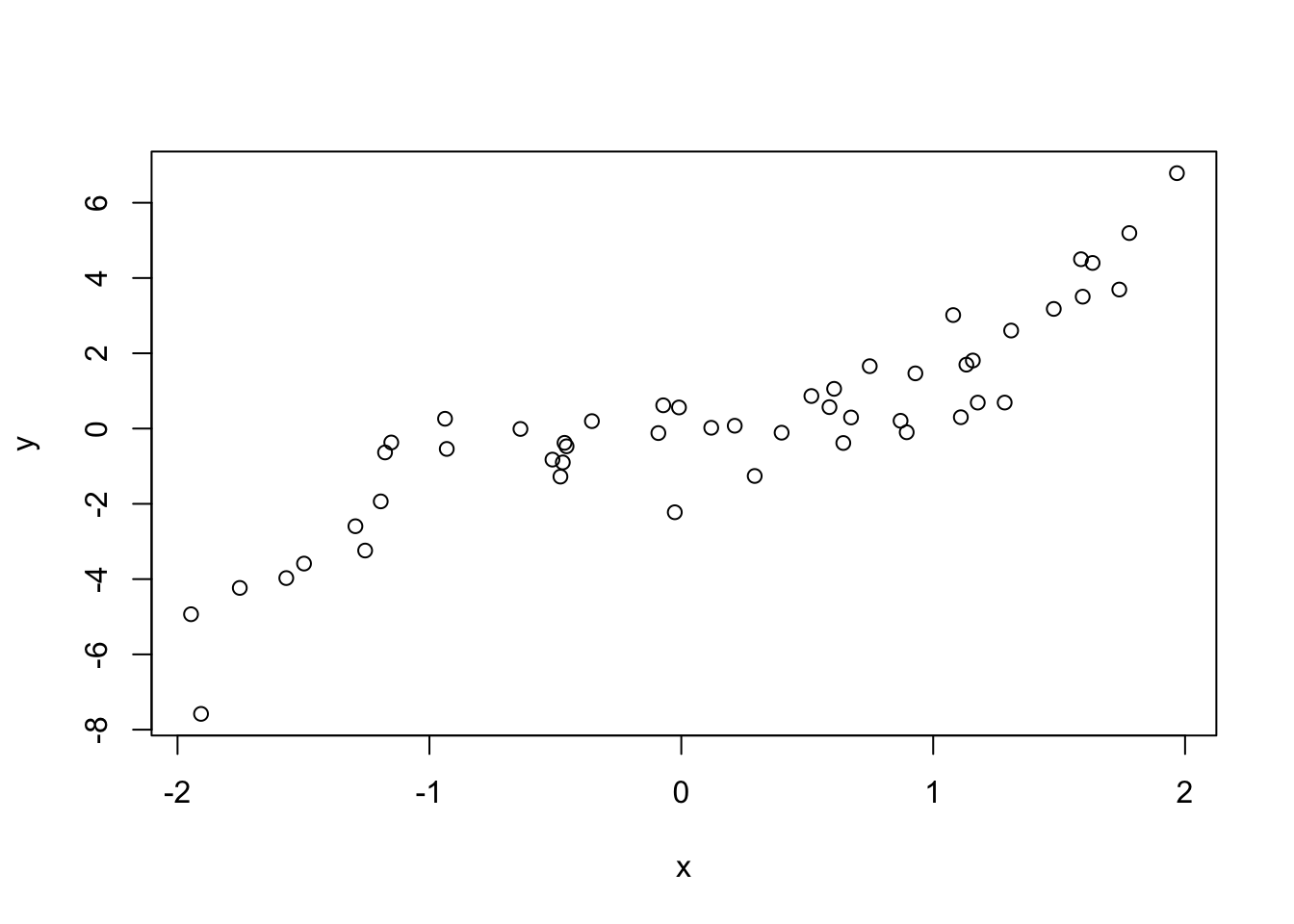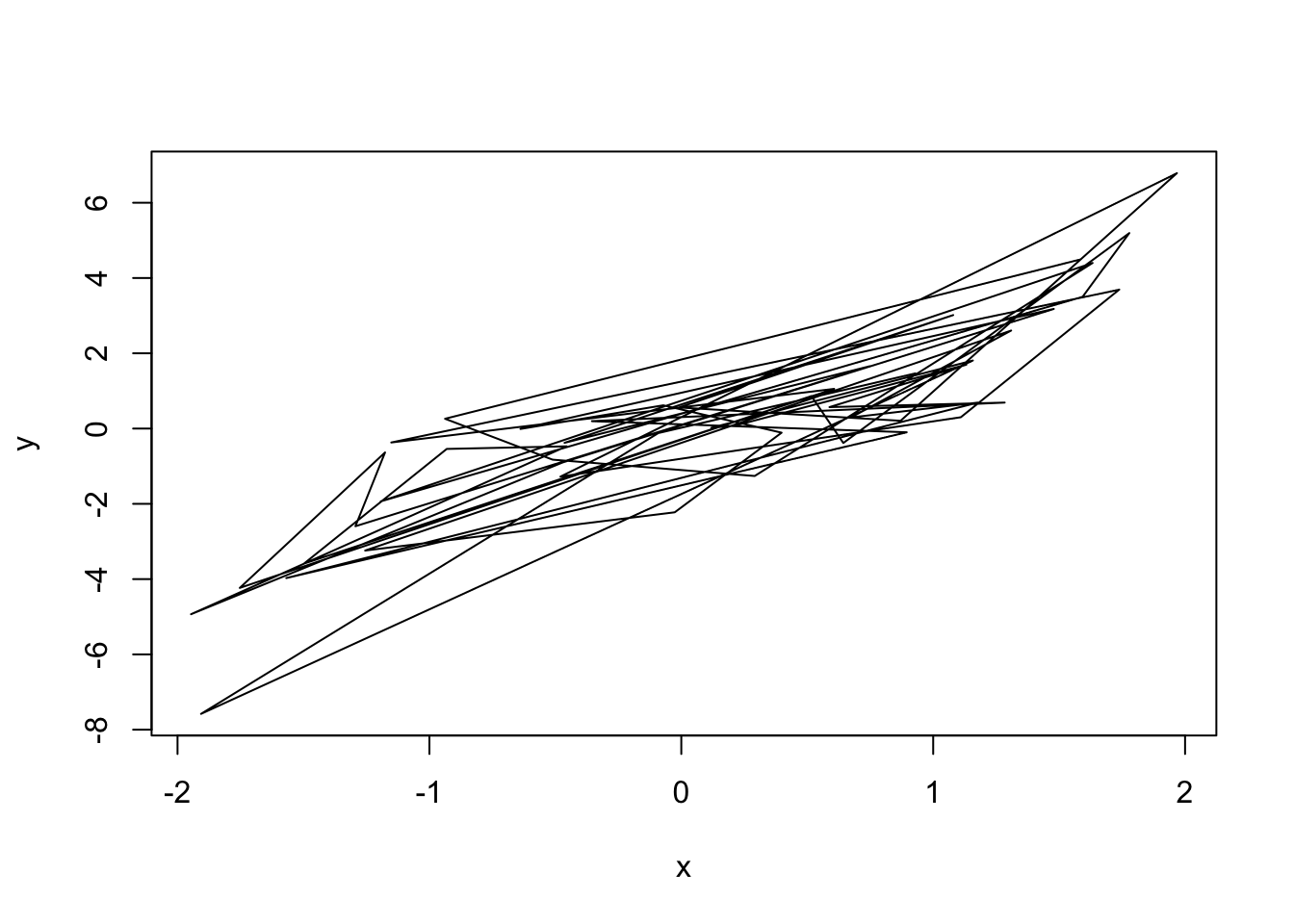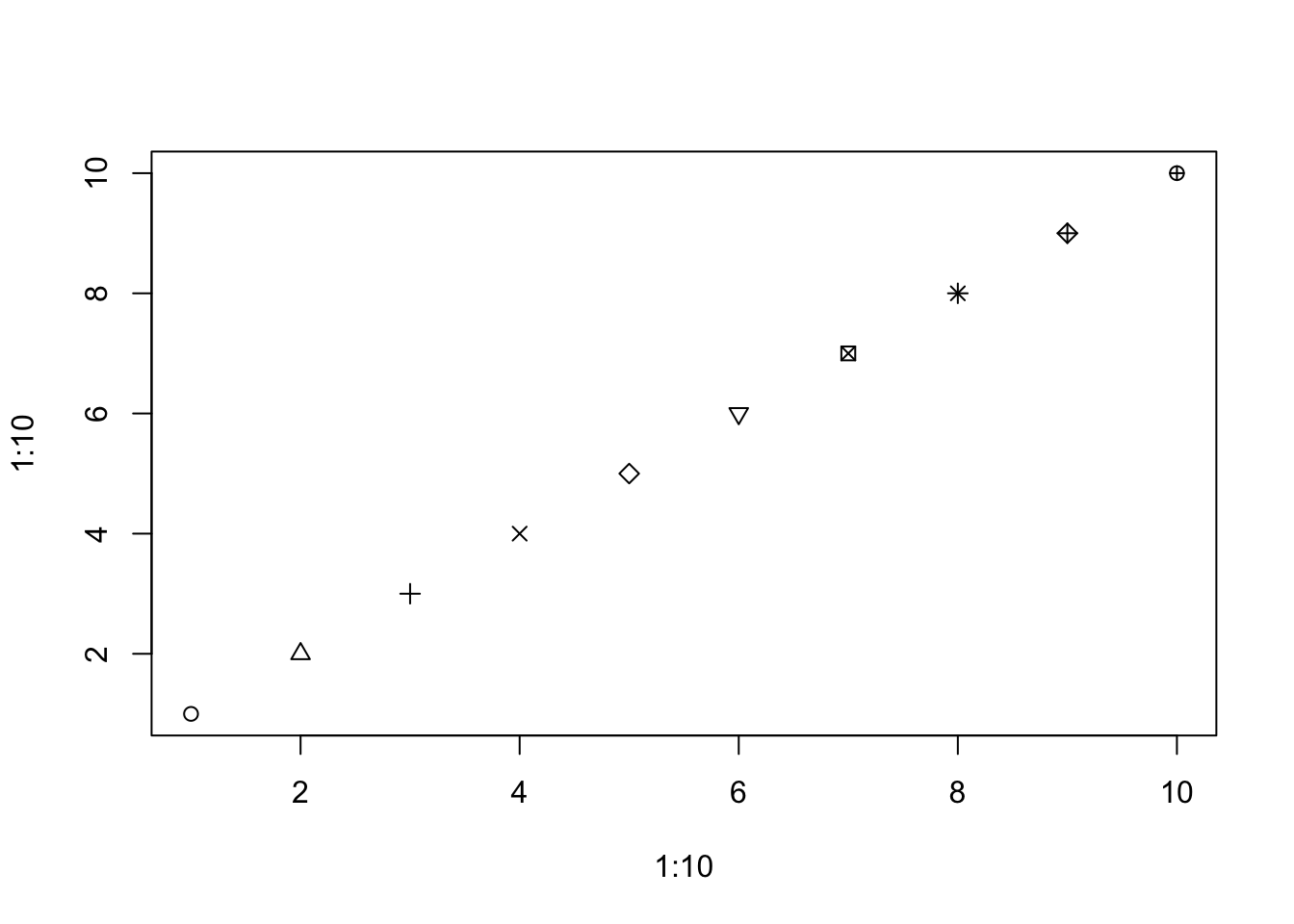Name:
Andrew ID:
Collaborated with:

This lab is to be done in class (completed outside of class time if need be). You can collaborate with your classmates, but you must identify their names above, and you must submit your own lab as an knitted PDF file on Gradescope, by Saturday 6pm, this week.

This week’s agenda: getting familiar with basic plotting tools; understanding the way layers work; recalling basic text manipulations; producing histograms and overlaid histograms; heatmaps.

# Q1. Plot basics

• 1a. Below is some code that is very similar to that from the lecture, but with one key difference. Explain: why does the plot() result with with type="p" look normal, but the plot() result with type="l" look abnormal, having crossing lines? Then modify the code below (hint: modify the definition of x), so that the lines on the second plot do not cross.
n = 50
set.seed(0)
x = runif(n, min=-2, max=2)
y = x^3 + rnorm(n)
plot(x, y, type="p")plot(x, y, type="l")# YOUR CODE GOES HERE
• 1b. The cex argument can used to shrink or expand the size of the points that are drawn. Its default value is 1 (no shrinking or expansion). Values between 0 and 1 will shrink points, and values larger than 1 will expand points. Plot y versus x, first with cex equal to 0.5 and then 2 (so, two separate plots). Give titles “Shrunken points”, and “Expanded points”, to the plots, respectively.
# YOUR CODE GOES HERE
• 1c. The xlim and ylim arugments can be used to change the limits on the x-axis and y-axis, repsectively. Each argument takes a vector of length 2, as in xlim = c(-1, 0), to set the x limit to be from -1 to 0. Plot y versus x, with the x limit set to be from -1 to 1, and the y limit set to be from -5 to 5. Assign x and y labels “Trimmed x” and “Trimmed y”, respectively.
# YOUR CODE GOES HERE
• 1d. Again plot y versus x, only showing points whose x values are between -1 and 1. But this time, define x.trimmed to be the subset of x between -1 and 1, and define y.trimmed to be the corresponding subset of y. Then plot y.trimmed versus x.trimmed without setting xlim and ylim: now you should see that the y limit is (automatically) set as “tight” as possible. Hint: use logical indexing to define x.trimmed, y.trimmed.
# YOUR CODE GOES HERE
• 1e. The pch argument, recall, controls the point type in the display. In the lecture examples, we set it to a single number. But it can also be a vector of numbers, with one entry per point in the plot. So, e.g.,

plot(1:10, 1:10, pch=1:10)displays the first 10 point types. If pch is a vector whose length is shorter than the total number of points to be plotted, then its entries are recycled, as appropriate. Plot y versus x, with the point type alternating in between an empty circle and a filled circle.

# YOUR CODE GOES HERE
• 1f. The col argument, recall, controls the color the points in the display. It operates similar to pch, in the sense that it can be a vector, and if the length of this vector is shorter than the total number of points, then it is recycled appropriately. Plot y versus x, and repeat the following pattern for the displayed points: a black empty circle, a blue filled circle, a black empty circle, a red filled circle.
# YOUR CODE GOES HERE

# Q2. Adding to plots

• 2a. Produce a scatter plot of y versus x, and set the title and axes labels as you see fit. Then overlay on top a scatter plot of y2 versus x2, using the points() function, where x2 and y2 are as defined below. In the call to points(), set the pch and col arguments appropriately so that the overlaid points are drawn as filled blue circles.
x2 = sort(runif(n, min=-2, max=2))
y2 = x^2 + rnorm(n)
# YOUR CODE GOES HERE
• 2b. Starting with your solution code from the last question, overlay a line plot of y2 versus x2 on top of the plot (which contains empty black circles of y versus x, and filled blue circles of y2 versus x2), using the lines() function. In the call to lines(), set the col and lwd arguments so that the line is drawn in red, with twice the normal thickness. Look carefully at your resulting plot. Does the red line pass overtop of or underneath the blue filled circles? What do you conclude about the way R layers these additions to your plot?
# YOUR CODE GOES HERE
• 2c. Starting with your solution code from the last question, add a legend to the bottom right corner of the the plot using legend(). The legend should display the text: “Cubic” and “Quadratic”, with corresponding symbols: an empty black circle and a filled blue circle, respectively. Hint: it will help to look at the documentation for legend().
# YOUR CODE GOES HERE
• 2d. Produce a plot of y versus x, but with a gray rectangle displayed underneath the points, which runs has a lower left corner at c(-2, qnorm(0.1)), and an upper right corner at c(2, qnorm(0.9)). Hint: use rect() and consult its documentation. Also, remember how layers work; call plot(), with type="n" or col="white" in order to refrain from drawing any points in the first place, then call rect(), then call points().
# YOUR CODE GOES HERE
• Challenge. Produce a plot of y versus x, but with a gray tube displayed underneath the points. Specifically, this tube should fill in the space between the two curves defined by $$y=x^3 \pm q$$, where $$q$$ is the 90th percentile of the standard normal distribution (i.e., equal to qnorm(0.90)). Hint: use polygon() and consult its documentation; this function requires that the x coordinates of the polygon be passed in an appropriate order; you might find it useful to use c(x, rev(x)) for the x coordinates. Lastly, add a legend to the bottom right corner of the plot, with the text: “Data”, “Confidence band”, and corresponding symbols: an empty circle, a very thick gray line, respectively.
# YOUR CODE GOES HERE

# Fastest 100m sprint times

Below, we read in two data sets of the 1000 fastest times ever recorded for the 100m sprint, in men’s and women’s track., as seen in previous labs.

sprint.m.df = read.table(
file="http://www.stat.cmu.edu/~ryantibs/statcomp/data/sprint.m.txt",
file="http://www.stat.cmu.edu/~ryantibs/statcomp/data/sprint.w.txt",
sep="\t", quote="", header=TRUE)

# Q3. Text manipulations, and layered plots

• 3a. Define sprint.m.times to be the first 4 characters of the Time column of sprint.m.df, and sprint.m.years to be the last 4 characters of the Date column of sprint.m.df. Hint: use substr(). Convert both to numeric vectors, and print the first 10 entries of each.
# YOUR CODE GOES HERE
• 3b. Plot sprint.m.times versus sprint.m.years. For the point type, use small, filled black circles. Label the x-axis “Year” and the y-axis “Time (seconds)”. Title the plot “Fastest men’s 100m sprint times”. Using abline(), draw a dashed blue horizontal line at 9.95 seconds. Using text(), draw below this line, in text on the plot, the string “N men”, replacing “N” here by the number of men who have run under 9.95 seconds. Your code should programmatically determine the correct number here, and use paste() to form the string. Comment on what you see visually, as per the sprint times across the years. What does the trend look like for the fastest time in any given year?
# YOUR CODE GOES HERE
• 3c. Reproduce the previous plot, but this time, draw a light blue rectangle underneath all of the points below the 9.95 second mark. The rectangle should span the entire region of the plot below the horizontal line at $$y=9.95$$. And not only the points of sprint times, but the blue dashed line, and the text “N men” (with “N” replaced by the appropriate number) should appear on top of the rectangle. Hint: use rect() and layering as appropriate.
# YOUR CODE GOES HERE
• 3d. Repeat Q3a but for the women’s sprint data, with one critical difference—to form the vector of times, take the first 5 characters of the Time column—and arrive at vectors sprint.w.times and sprint.w.years. Then repeat Q3c for this data, but with the 9.95 second cutoff being replaced by 10.95 seconds, the rectangle colored pink, and the dashed line colored red. Comment on the differences between this plot for the women and your plot for the men, from Q4c. In particular, is there any apparent difference in the trend for the fastest sprint time in any given year?
# YOUR CODE GOES HERE

# Q4. More text manipulations, and histograms

• 4a. Extract the birth years of the sprinters from the data frame sprint.m.df. To do so, define a character vector sprint.m.byears to contain the last 2 characters of the Birthdate column of sprint.m.df. Then convert sprint.m.byears into a numeric vector, add 1900 to each entry, and redefine sprint.m.byears to be the result. Repeat the same, but for the women’s data, arriving at a vector called sprint.w.byears.
# YOUR CODE GOES HERE
• 4b. What are the ranges of birth years you computed in the last part, for the men’s and women’s data? Do you see any problems with this? You should! Fix the errant entries in sprint.m.byears and sprint.w.byears using simple indexing and arithmetic. Hint: none of these athletes were born before 1921.
# YOUR CODE GOES HERE
• 4c. Now that you have fixed their birthdates, create a vector sprint.m.ages containing the age (in years) of each male sprinter when their sprint time was recorded. Do the same for the female sprinters, resulting in sprint.w.ages. Hint: use sprint.m.years and sprint.w.years.
# YOUR CODE GOES HERE
• 4d. Using one of the apply functions, compute the average sprint time for each age in sprint.m.ages, calling the result time.m.avg.by.age. Similarly, compute the analogous quantity for the women, calling the result time.w.avg.by.age. Are there any ages for which the men’s average time is faster than 9.9 seconds, and if so, which ones? Are there any ages for which the women’s average time is faster than 10.9 seconds, and if so, which ones?
# YOUR CODE GOES HERE
• 4e. Plot a histogram of sprint.m.ages, with break locations occuring at every age in between 17 and 40. Color the histogram to your liking; label the x-axis, and title the histogram appropriately. What is the mode, i.e., the most common age? Also, describe what you see around the mode: do we see more sprinters who are younger, or older?
# YOUR CODE GOES HERE
• Challenge. Plot a histogram of sprint.m.ages, now with probability=TRUE (so it is on the probability scale, rather than raw frequency scale). Overlay a histogram of sprint.w.ages, also with probability=TRUE. Set the break locations so that the plot captures the full range of the very youngest to the very oldest sprinter present among both men and women. Your code should determine these limits programmatically. Choose colors of your liking, but use transparency as appropriate so that the shapes of both histograms are visible; label the x-axis, and title the histogram appropriately. Add a legend to the histogram, identifying the histogram bars from the men and women. Compare, roughly, the shapes of the two histograms: is there a difference between the age distributions of the world’s fastest men and fastest women?
# YOUR CODE GOES HERE

# Q5. Maungawhau volcano and heatmaps (optional)

• Challenge. The volcano object in R is a matrix of dimension 87 x 61. It is a digitized version of a topographic map of the Maungawhau volcano in Auckland, New Zealand. Plot a heatmap of the volcano using image(), with 25 colors from the terrain color palette.
# YOUR CODE GOES HERE
• Challenge. Each row of volcano corresponds to a grid line running east to west. Each column of volcano corresponds to a grid line running south to north. Define a matrix volcano.rev by reversing the order of the rows, as well as the order of the columns, of volcano. Therefore, each row volcano.rev should now correspond to a grid line running west to east, and each column of volcano.rev a grid line running north to south.
# YOUR CODE GOES HERE
• Challenge. If we printed out the matrix volcano.rev to the console, then the elements would follow proper geographic order: left to right means west to east, and top to bottom means north to south. Now, produce a heatmap of the volcano that follows the same geographic order. Hint: recall that the image() function rotates a matrix 90 degrees counterclockwise before displaying it; and recall the function clockwise90() from the lecture, which you can copy and paste into your code here. Label the x-axis “West –> East”, and the y-axis “South –> North”. Title the plot “Heatmap of Maungawhau volcano”.
# YOUR CODE GOES HERE
• Challenge. Reproduce the previous plot, and now draw contour lines on top of the heatmap.
# YOUR CODE GOES HERE
• Challenge. The function filled.contour() provides an alternative way to create a heatmap with contour lines on top. It uses the same orientation as image() when plotting a matrix. Use filled.contour() to plot a heatmap of the volcano, with (light) contour lines automatically included. Make sure the orientation of the plot matches proper geographic orientation, as in the previous question. Use a color scale of your choosing, and label the axes and title the plot appropriately. It will help to consult the documentation for filled.contour().
# YOUR CODE GOES HERE